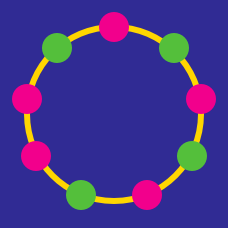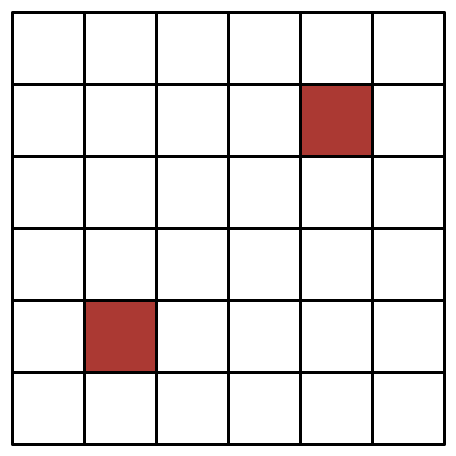Probability

# Discrete Mathematics Warmups: Level 5 ChallengesWhat is the probability (to 3 decimal places) that a point $P$ picked uniformly at random inside an equilateral triangle, is closer to the centroid than to its sides?

Consider the set $\mathbb{S} =\{B,R,I,L,A,N,T\}$. How many ways are there for us to partition the set into any number of non empty disjoint subsets whose Union is $\mathbb{S}$?.

Suppose two real numbers $x$ and $y$ are chosen, uniformly and at random, from the open interval $(0,1)$. Let $P$ be the probability that the integer closest to $\dfrac{x}{y}$ is odd.

Find $\lceil 10000P \rceil$.

Let $a_0, a_1, \cdots, a_7$ be any $8$ distinct integers. Let $P$ be the product of their pairwise differences, that is:

$P = \prod _ {i < j} {(a_i - a_j)}$

What is the greatest integer which always divides $P?$In the above image, how many rectangles are there which do not include any red squares?

×

Problem Loading...

Note Loading...

Set Loading...